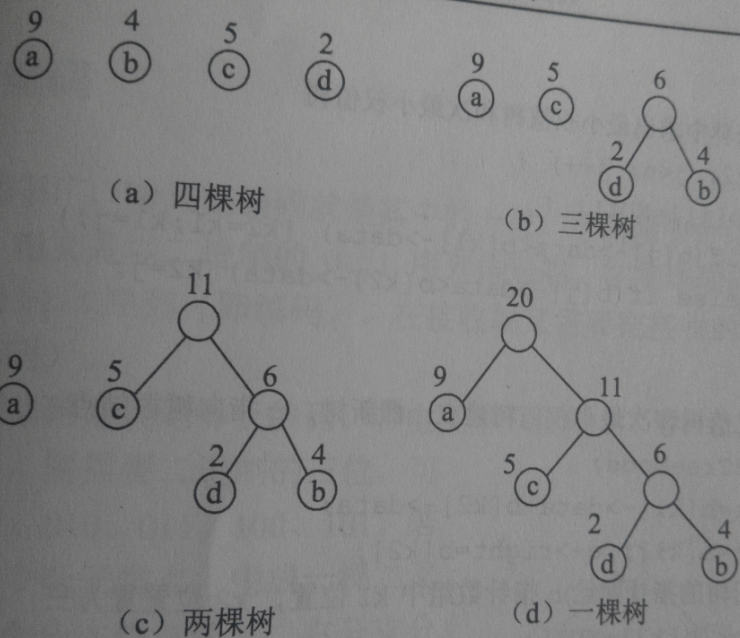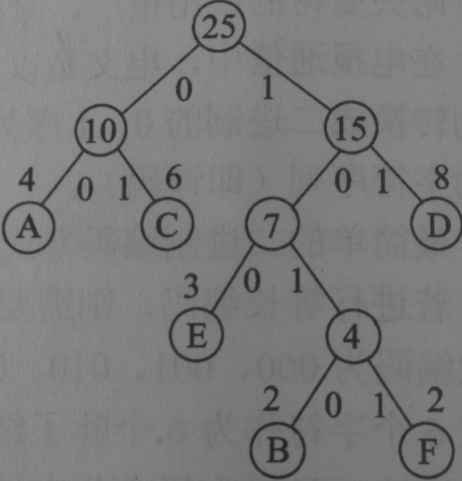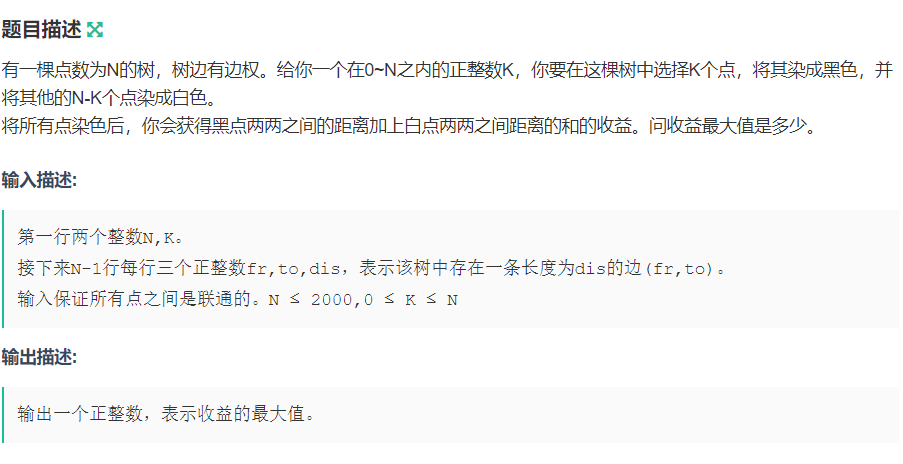• WPL（带权最优路径）怎么算？   WPL等于每个点的*它所在的层数相加 怎么构造哈夫曼？  有n个点，并且知道这n个点的，先选出最小的的两个点，合并出一个父节点，再由这个父节点和剩下的最小的的点再合并...
哈夫曼树又称最优二叉树
先说一下权是什么意思？
权就是每个节点上有一个实数，这个实数就代表这个点的权
WPL（带权最优路径）怎么算？
WPL等于每个点的权*它所在的层数相加
怎么构造哈夫曼树？
有n个点，并且知道这n个点的权，先选出最小的权的两个点，合并出一个父节点，再由这个父节点和剩下的最小的权的点再合并出一个新的父节点当做新的一个点，如果有两个点的权都小于这个父节点，则在旁边再合并成一个父节点当做一个点再运算，尽量权小的点合并，直到所有点都在这颗树上哈夫曼编码
由编码哈夫曼树得到的字符编码称作哈夫曼编码
哈夫曼编码就是右子树为1，左子树为0
给个例子：A B C D E F 的编码分别为00 ， 1010，  01，  11，  100，  1011


展开全文java
• 给定一棵nnn个点带边，你可以删去其中KKK条边，再连上KKK条边权为000的边，价值是大路径。求所有方案中你能得到的最大价值。 n,m≤3×105n,m\leq 3\times 10^5n,m≤3×105 【解题思路】 考虑已经删掉了边，...
update
为什么CSDN这么辣鸡？我戳进来又要我再发表一次？然后时间就不对了。这个题我之前就写了。
【题目】
LOJ
给定一棵$n$个点带边权的树，你可以（且必须）删去其中$K$条边，再连上$K$条边权为$0$的边，价值是大路径权。求所有方案中你能得到的最大价值。
$n,K\leq 3\times 10^5$
【解题思路】
考虑已经删掉了边，答案在树中应该是怎么样的？显然是由原树中恰好$K+1$条点不相交的链组成的（单独一个点也算）。
现在问题就是在树上选择$K$条点不相交的链使得边权和最大。
不妨设$f_{i,j,0/1/2}$表示在$i$这个点，子树中选择了$j$条链，这个点和子树中多少条链相连，的最大权值和。那么转移就是一个类似背包的东西，复杂度$O(nk^2)$。
观察到答案关于$K$的函数应该是一个单峰的函数，不妨考虑进行带权二分。
具体来说，我们二分一个斜率$s$，每次新选择一条路径时，需要额外失去$s$点权值，要求的就是选择的最大权值和是多少。
这个问题我们可以在$O(n)$的时间内用一个简单的$\text{DP}$求解，方式和之前的做法一样，只是少了$K$这一维。
这样做复杂度是$O(n\log V)$的。
$\text{DP}$的时候我们需要记录最优的时候至少选择几条链，但由于答案函数可能在一段区间中都取到最优值，无法恰好二分出$K$条链，不过没有关系，我们一定能构造出来答案，那么需要保留的就是最接近$K$且不超过$K$的答案。
一些细节：二分的时候只需要在整数范围内二分就可以了，选择单独一个根节点时，看作与子树中$1$条链相连，更新时倒序更新。
一个警告：不要使用pair类型重载大于小于号，否则很有可能出现问题。（下面的代码懒得改了，虽然这样写是对的）
【参考代码】
#include<bits/stdc++.h>
#define fi first
#define se second
#define mkp make_pair
using namespace std;

typedef long long ll;
typedef pair<int,ll>pii;//times,val
const ll inf=1e15;
const int N=3e5+10;
int n,K,tot,head[N];
ll mid,ans,rem;
pii f[N];

int read()
{
int ret=0,f=1;char c=getchar();
while(!isdigit(c)) {if(c=='-')f=0;c=getchar();}
while(isdigit(c)) ret=ret*10+(c^48),c=getchar();
return f?ret:-ret;
}

struct Tway{int v,nex,w;}e[N<<1];
void add(int u,int v,int w)
{
e[++tot]=(Tway){v,head[u],w};head[u]=tot;
e[++tot]=(Tway){u,head[v],w};head[v]=tot;
}

bool operator <(const pii&a,const pii&b){return a.se==b.se?a.fi>b.fi:a.se<b.se;}
pii Max(const pii&a,const pii&b){return a<b?b:a;}
pii operator +(const pii&a,const pii&b){return mkp(a.fi+b.fi,a.se+b.se);}
pii operator +(const pii&a,const ll&b){return mkp(a.fi,a.se+b);}
void gmax(pii&a,pii b,int c){b.fi+=c;a=Max(a,b);}

void dfs(int x,int fa)
{
f[x]=mkp(0,0);f[x]=mkp(1,-mid);f[x]=mkp(0,-inf);
for(int i=head[x];i;i=e[i].nex)
{
int v=e[i].v,w=e[i].w;
if(v==fa) continue; dfs(v,x);
pii a=Max(f[v],Max(f[v],f[v]));
//printf("%d %lld\n",a.fi,a.se);
gmax(f[x],f[x]+a,0);gmax(f[x],f[x]+f[v]+mid+w,-1);
gmax(f[x],f[x]+a,0);gmax(f[x],f[x]+f[v]+w,0);
gmax(f[x],f[x]+a,0);
}
}

int solve()
{
dfs(1,0);pii res=mkp(0,-inf);
for(int i=1;i<=n;++i) res=Max(res,Max(f[i],Max(f[i],f[i])));
if(res.fi<=K) ans=res.se;
//printf("%lld %d %lld\n",mid,res.fi,res.se);
return res.fi;
}

int main()
{
#ifndef ONLINE_JUDGE
freopen("LOJ2478.in","r",stdin);
freopen("LOJ2478.out","w",stdout);
#endif
n=read();K=read()+1;
for(int i=1,x,y;i<n;++i) x=read(),y=read(),add(x,y,read());
ll l=-1e12,r=1e12;
while(l<=r)
{
mid=(l+r)>>1;
if(solve()<=K) rem=mid,r=mid-1;
else l=mid+1;
}
printf("%lld\n",ans+rem*K);
return 0;
}



展开全文• 无向连通带权图或有向连通带权图里面的怎么算的啊，就像下面的图![图片](https://img-ask.csdn.net/upload/201511/24/1448369024_640499.jpg)离散数学
• Description给定一颗n个结点的无根上的每个点有一个非负整数点，定义一条路径的价值为路径上的点和-路径的点最大值。 给定参数p，我们想知道，有多少不同的上简单路径，满足它的价值恰好是p的倍数。 ...
Description

给定一颗n个结点的无根树，树上的每个点有一个非负整数点权，定义一条路径的价值为路径上的点权和-路径的点权最大值。
给定参数p，我们想知道，有多少不同的树上简单路径，满足它的价值恰好是p的倍数。
注意：单点算作一个路径；u ≠ v时，（u,v）和（v,u）只算一次。

Solution

首先套上树分治模板，再想想怎么做……
可以发现，若是想满足值为p的倍数，那就意味着需要该值mod p=0，问题来了，如何统计一个子树的答案呢？其实很容易解决，可以发现，当一条链的贡献是x时，它需要另一条贡献为（p-x）的链结合（在模p意义下），那么我们可以开一个桶来储存，对于减去的最大值，我们只需要把每一条链按最大值排个序，就能够保证每一次减去的最大值是当前链的最大值。还有要记得去重的问题，因为每一棵子树都会被算多，在做完当前树之后要减去每棵子树的贡献。时间复杂度为（n log n）。

Code

#include<iostream>
#include<algorithm>
#include<stdio.h>
#include<string.h>
#include<math.h>
using namespace std;
#define fo(i,a,b) for(i=a;i<=b;i++)
#define fd(i,a,b) for(i=a;i>=b;i--)
#define rep(i,x) for(i=la[x];i;i=ne[i])
typedef long long ll;
const int N=1e5+5,MX=1e7+5;
struct arr{
ll s;int mx;
}t[N];
int la[N],ne[N*2],da[N*2],node[N],T[MX],D[N],fa[N],a[N];
int n,mo,i,x,y,sum,num,hea;
ll ans;
bool p[N],bz[N];
void ins(int x,int y){
da[++sum]=y,ne[sum]=la[x],la[x]=sum;
da[++sum]=x,ne[sum]=la[y],la[y]=sum;
}
bool cmp(arr x,arr y){return x.mx<y.mx;}
void gnode(int x){
int i,l=0,r=1;D=x;fa[x]=0;
while(l<r){
x=D[++l];
rep(i,x) if(da[i]!=fa[x]&&!p[da[i]]) D[++r]=da[i],fa[da[i]]=x;
}l++;
while(l>1){
x=D[--l];node[x]=1;
rep(i,x) if(da[i]!=fa[x]&&!p[da[i]]) node[x]+=node[da[i]];
}
}
void gheav(int x,int size){
int i,l=0,r=1;bz[x]=1;D=x;fa[x]=0;
while(l<r){
x=D[++l];
rep(i,x) if(da[i]!=fa[x]&&!p[da[i]]){
if(node[da[i]]>size/2) bz[x]=0;
D[++r]=da[i];bz[da[i]]=1;fa[da[i]]=x;
}
}l++;
while(l>1){
x=D[--l];if(bz[x]&&size-node[x]<=size/2) hea=x;
}
}
void dfs(int x,int fa,ll sum,int mx){
t[++num]=(arr){sum,mx};
int i;rep(i,x) if(da[i]!=fa&&!p[da[i]]) dfs(da[i],x,sum+a[da[i]],max(mx,a[da[i]]));
}
void deal(int tot,int x,ll sum,int mx,int zf){
num=0;dfs(x,0,sum,mx);
sort(t+1,t+num+1,cmp);
fo(i,1,num){
ans+=T[(t[i].s-t[i].mx)%mo]*zf;
T[((mo-t[i].s+tot)%mo+mo)%mo]++;
}
fo(i,1,num)T[((mo-t[i].s+tot)%mo+mo)%mo]--;
}
void divi(int x){
int i;
gnode(x);hea=0;gheav(x,node[x]);x=hea;
p[x]=1;deal(a[x],x,a[x],a[x],1);
rep(i,x) if(!p[da[i]]){
deal(a[x],da[i],a[x]+a[da[i]],max(a[x],a[da[i]]),-1);
divi(da[i]);
}
}
int main(){
freopen("path.in","r",stdin);
freopen("path.out","w",stdout);

scanf("%d%d",&n,&mo);
fo(i,2,n) scanf("%d%d",&x,&y),ins(x,y);
fo(i,1,n) scanf("%d",&a[i]);
divi(1);ans+=n;
printf("%lld",ans);
}
展开全文• 接下来就是考虑怎么去计算出收益，因为不能暴力枚举黑点距离，所以我们可以考虑每条边的贡献， 贡献=(子树内黑点 ⋅\cdot⋅ 子树外黑点+子树内白点 ⋅\cdot⋅ 子树外白点) ⋅\cdot⋅ 边 转移方程就是类似于背包...
题目链接
题面：题解：
设$sz[i]$ 为以$i$为根的子树的大小，设$dp[i][j]$ 为以$i$ 为根的子树，选$j$ 个点染成黑色的最大收益。
接下来就是考虑怎么去计算出收益，因为不能暴力枚举黑点算距离，所以我们可以考虑每条边的贡献，
贡献=(子树内黑点 $\cdot$ 子树外黑点+子树内白点 $\cdot$ 子树外白点) $\cdot$ 边权
转移方程就是类似于背包，枚举当前黑点数和子树的黑点数
代码：
#include<cstdio>
#include<iostream>
#include<algorithm>
#include<cstring>
#include<cmath>
#include<queue>
#include<map>
#include<stack>
#include<set>
#include<ctime>
#define iss ios::sync_with_stdio(false)
using namespace std;
typedef unsigned long long ull;
typedef long long ll;
const int mod=1e9+7;
const int MAXN=2e3+5;
const ll inf=0x3f3f3f3f3f;
struct node
{
int to;
int next;
ll cost;
}e[MAXN<<1];
int cnt,n,k;
int head[MAXN];
int sz[MAXN];
ll dp[MAXN][MAXN];
void add(int u,int v,int w){
e[cnt].to=v;
e[cnt].next=head[u];
e[cnt].cost=w;
head[u]=cnt++;
}
void dfs(int u,int f)
{
dp[u]=dp[u]=0;
sz[u]=1;
for(int i=head[u];i!=-1;i=e[i].next)
{
int v=e[i].to;
if(v==f) continue;
dfs(v,u);
sz[u]+=sz[v];
for(int j=min(k,sz[u]);j>=0;j--)
{
for(int z=0;z<=min(j,sz[v]);z--)
{
ll cnt=z*(k-z)+(sz[v]-z)*(n-k-sz[v]+z);
dp[u][j]=max(dp[u][j],dp[u][j-z]+dp[v][z]+cnt*e[i].cost);
}
}
}
}
int main()
{
memset(dp,-inf,sizeof dp);
memset(head,-1,sizeof  head);
scanf("%d%d",&n,&k);
for(int i=1;i<=n-1;i++){
int u,v;ll w;
scanf("%d%d%lld",&u,&v,&w);
add(u,v,w);
add(v,u,w);
}
dfs(1,-1);
printf("%lld\n",dp[k]);
}




展开全文• 2.那把问题转化一下， 他要求所有黑点到白点的最小距离，我们可以求一颗生成每条边的贡献时 只要计算出这条边两侧黑点数和白点数分别是多少就好了（就可以出这条边走了几次）。 那怎么才能是
• Tree 题目链接 题目大意 给一棵，让选择一个联通的子图 满足: 度数大于k的点的数量不超过1个（小于等于1） 图是联通的。...dp[x]怎么算？ 有两种情况： 当这个度数大于k的点是x的时候，dp[x]
• 假设我们一个权重为1,7,3,13,12,15,24怎么样画出哈夫曼和计算带权路径长度。 首先，选出最小的两个重值，这里是1,3（矩形表示叶子节点，圆表示根节点也是两个叶子节点的和）如图： 然后，选出第三小的7，...
• 有个结论：相同边的边集不论怎么选，对最小生成树的贡献都相同（选的数量都会相同，而且连完之后图的连通性也会相同） 所以可以把不同边的边分开来看，答案就是每种边的方案之积。 于是就可以愉快地暴力了： ...
• 一棵有边，问有多少对点的简单路径权值和模3为0 思路： 简单的分治，每次处理出重心后，处理出到重心的距离，然后为0的直接平方，1和2的相乘并乘2（因为（2，3），（3，2）两对），然后删去不合法的...
• 不过BJ对kruskal重构也仅仅理解 没写过。。 希望有人带带 QWQ 告诉我对不对、怎么做哦~ LCT维护最大生成 加入一条边时 若两点未联通 直接加 否则找到两点路径上最小的边 看能不能替换掉 #include #...
• LINK nnn个点的,点iii的点是aia_iai​ 求∑u=1n∑v=1nmin(au,av)∗dis(u,v)\sum\limits_{u=1}^n\sum\limits_{v=1}^n\rm min(a_u,a_v)*dis(u,v)u=1∑n​v=1...通过点uuu的路径贡献怎么算… 先爆搜出所有从uuu出发的
• jQuery是目前流行的JS封装包，简化了很多复杂的JS程序，JQuery讲浏览器DOM定义为$，通过$来获取各个子节点。JS插件还有prototype.js 等，它们也比较好的插件，也使用\$。所以有时候同时使用这个两个JS插件的时候...
• 上的路径的价值定义为上路径的点和-上路径的点最大值; 现在给定一个参数P询问有多少条路径的价值是P的倍数(注意单点也路径,路径不重复) 这个题一眼就知道是点分治之类的鬼东西,第一眼觉得长得想聪...
• 这题打比赛的时候只想到了质数怎么分配的，没有想到边的贡献怎么算，后来看了大佬的blog才知道的，Orz，其实你把一个找个顶点dfs一遍，设size[i]为该结点子的大小，那么该点与其父亲结点的边的贡献为size[i]⋅(n...
• 首先huffman编码长度怎么算呢。。其实就是以每种字符出现的次数为点，建一棵huffman，边权为1，它的WPL其实就是这段字符huffman编码的长度。所以我们就用莫队维护一下每个字符出现的次数啦，然后模拟建huffman...
• 二分查找法（在有序数组中查找某一特定元素的搜索算法）3.DFS（深度优先搜索，沿着的深度遍历的节点）4.Dijkstra算法（用广度优先搜索解决非负有向图的单源最短路径问题，算法最终得到一个最短路径怎么...遗传算法
• 那么怎么统计呢，我们对于每个点赋一个点，方点点为点双的大小，圆点点权为$$-1$$。 那么这条路径的点和就是答案，注意要统计到端点的权值。 然后优化就很显然了，直接枚举每个点被了多少次就行了，这个随便...
• [思路] 对权值离散化后（要先读入所有输入里的权值一起离散化……所以一共有4e4个数据（~~当然你也可以不读入 hehe~~~~）） 建立一颗线段， 线段单点维护每一个海拔下的答案 好了问题来了， 怎么算答案呢？...
• LCT 最小生成 洛谷题目传送门 BZOJ题目传送门 改了好久。。。都跨月了 最后发现x&...注意数据加强版要先在最终状态的那几条边跑MST，完成了就break，不能像那道题一样和加边一起（别问我怎么知道的，1...
• 第3节 Bellman-Ford——解决负边 163 第4节 Bellman-Ford的队列优化 171 第5节 zui短路径算法对比分析 177 第7章 神奇的 178 第1节 开启“”之旅 179 第2节 二叉树 183 第3节 堆——神奇的优先队列 ...C语言
• 水平当然也有限，不过对于新手入门，绝对没问题，如果你还不会，那我输！！ 视频怎么看？ 理论科班出身-建议去学习 Andrew Ng 的视频（Ng 的视频绝对是权威，这个毋庸置疑） 编码能力强 - 建议看我们的《机器...
• 水平当然也有限，不过对于新手入门，绝对没问题，如果你还不会，那我输！！ 视频怎么看？ 理论科班出身-建议去学习 Andrew Ng 的视频（Ng 的视频绝对是权威，这个毋庸置疑） 编码能力强 - 建议看我们的《机器...
• 水平当然也有限，不过对于新手入门，绝对没问题，如果你还不会，那我输！！ 视频怎么看？ 理论科班出身-建议去学习 Andrew Ng 的视频（Ng 的视频绝对是权威，这个毋庸置疑） 编码能力强 - 建议看我们的《机器...adaboost apriori fp-growth